# Writing grid Extensions

New hook functions, makeContext() and makeContent(), have been added to the grid graphics package. These functions allow an alternative approach to developing custom grobs when a grob can only decide what to draw at drawing time rather than when the grob is created. For custom grobs that are based on this new approach, the grid.force() function provides access to low-level grobs that are otherwise invisible because they are only generated at drawing time. These functions lead to greater flexibility in the development of custom grobs and more power to modify the result after drawing is complete.

From R 2.16.0 there is a new recommended approach to developing customised grid grobs. In a nutshell, two new "hook" functions, makeContext() and makeContent() have been added to grid to provide an alternative to the existing hook functions preDrawDetails(), drawDetails(), and postDrawDetails(). This article provides a simple demonstration of the use of these new functions and discusses the benefits that accrue from this new approach.

## A simple grid customisation

In order to demonstrate the development of a custom grid grob, we will consider several different ways to produce a "boxed label" with grid. This grob will draw a text label and surround the text with a box (with rounded corners). The simplest way to implement this sort of thing in grid is to write a function that makes several calls to draw existing grid grobs. For example, the following code defines a textbox() function that takes a single argument, a text label, and calls grid.text() to draw the label and then grid.roundrect() to draw a box around the label. The stringWidth() and stringHeight() functions are used to make sure that the box is the right size for the label.
```> library(grid)
```
```> textbox <- function(label) {
+     grid.text(label, name="text")
+     grid.roundrect(width=1.5*stringWidth(label),
+                    height=1.5*stringHeight(label),
+                    name="box")
+ }
```
The following code shows the function in action and the output is shown below the code.
```> grid.newpage()
> textbox("test")
```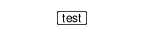This function has produced two grobs, a text grob and a roundrect grob.
```> grid.ls(fullNames=TRUE)
```
```text[text]
roundrect[box]
```
However, there is no connection between these grobs. For example, if we modify the text (code below), the roundrect stays the same size and becomes too small for the text (see the output below the code).
```> grid.edit("text", label="hello world")
```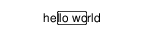It is possible to group the two grobs together by constructing a gTree to contain them both. For example, the following code redefines the textbox() function so that it generates a gTree containing a text grob and a roundrect grob and then draws the gTree.
```> textbox <- function(label) {
+     tg <- textGrob(label, name="text")
+     rr <- roundrectGrob(
+               width=1.5*stringWidth(label),
+               height=1.5*stringHeight(label),
+               name="box")
+     grid.draw(gTree(children=gList(tg, rr),
+                     name="tb"))
+ }
```
This version of the function produces the same output as the previous version.
```> grid.newpage()
> textbox("test")
```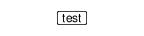However, the scene now consists of a single gTree that contains the text grob and the roundrect grob.
```> grid.ls(fullNames=TRUE)
```
```gTree[tb]
text[text]
roundrect[box]
```
But the contents of the gTree are fixed at creation time, so if we modify the text grob child of the gTree, the roundrect child is still not updated.
```> grid.edit("tb::text", label="hello world")
```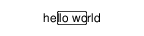## The old drawDetails() hook

The behaviour of the text box can be made more coherent if we delay the construction of the box until drawing time (i.e., recalculate the box every time that we draw). This can be achieved in grid by creating a custom grob and then defining a drawDetails() method for the custom grob. For example, the following code redefines the textbox() function so that it generates a custom "textbox" grob and draws that.
```> textbox <- function(label,
+                     name=NULL, gp=NULL, vp=NULL) {
+     grid.draw(grob(label=label,
+                    name=name, gp=gp, vp=vp,
+                    cl="textbox"))
+ }
```
For this function to draw anything, we also need to define a drawDetails() method for "textbox" grobs. Such a method is shown in the code below, which is almost identical to the first version of textbox(); all that we have done is delay the generation of the text and box until drawing time.
```> drawDetails.textbox <- function(x, ...) {
+     grid.text(x\$label, name="text")
+     grid.roundrect(
+         width=1.5*stringWidth(x\$label),
+         height=1.5*stringHeight(x\$label),
+         name="box")
+ }
```
The following code shows the new textbox() function in action and shows that it produces exactly the same output as the first version.
```> grid.newpage()
> textbox("test", name="tb")
```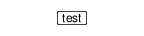One big difference is that only one "textbox" grob was generated, rather than separate text and roundrect grobs. The latter are only generated at drawing time and are not retained.
```> grid.ls(fullNames=TRUE)
```
```textbox[tb]
```
Another big difference is that, if we modify that one grob, both the text and the box are updated.
```> grid.edit("tb", label="hello world")
```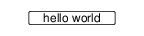However, one problem with this solution is that the text and box are no longer visible as separate grobs. For example, it is possible to change both text and box to grey (as below), but it is not possible to affect either just the text or just the box separately.
```> grid.edit("tb", gp=gpar(col="grey"))
```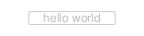In other words, we have a convenient high-level interface to the combined text and box, but we only have that high-level interface.

## The new makeContent() hook

The new makeContent() function provides an alternative way to develop a custom grid grob. The main difference is that, whereas a drawDetails() method typically calls grid functions to draw output, a makeContent() method calls grid functions to generate grobs. The standard behaviour for grobs automatically takes care of drawing the content. To continue our example, the following code redefines textbox() yet again. This is almost identical to the previous version of textbox(). The one important difference here is that the gTree() function is used to generate a custom gTree, rather than calling the grob() function to generate a simple custom grob. This is necessary whenever a makeContent() method generates more than one grob. We also create a different class than before, called "textboxtree" so that we do not have both drawDetails() and makeContent() methods defined for the same class.
```> textbox <- function(label,
+                     name=NULL, gp=NULL, vp=NULL) {
+     grid.draw(gTree(label=label,
+                     name=name, gp=gp, vp=vp,
+                     cl="textboxtree"))
+ }
```
So that this custom gTree will draw something, we define a makeContent() method. This is similar to the drawDetails() method above because it generates a text grob and a roundrect grob, but it does not draw them, it simply adds these grobs as children of the gTree. The modified gTree must be returned as the result of this function so that grid can draw the generated content.
```> makeContent.textboxtree <- function(x) {
+     t <- textGrob(x\$label,
+                   name="text")
+     rr <- roundrectGrob(width=1.5*grobWidth(t),
+                         height=1.5*grobHeight(t),
+                         name="box")
+     setChildren(x, gList(t, rr))
+ }
```
The following code shows that the new textbox() function produces exactly the same output as before.
```> grid.newpage()
> textbox("test", name="tbt")
```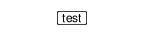As with the drawDetails() approach, the scene consists of only one grob, this time a "textboxtree" grob.
```> grid.ls(fullNames=TRUE)
```
```textboxtree[tbt]
```
Furthermore, if we modify that one grob, both the text and the box are updated.
```> grid.edit("tbt", label="hello world")
```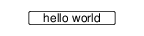In summary, the makeContent() approach behaves exactly the same as the drawDetails() approach. The advantages of the makeContent() approach lie in the extra things that it allows us to do.

## The grid.force() function

The new function grid.force() affects any custom grobs that have a makeContent() method. This function replaces the original grob with the modified grob that is returned by the makeContent() method. For example, if we use grid.force() on a scene that contains a "textboxtree" grob, the output of the scene is unaffected (see below).
```> grid.force()
```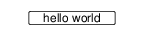However, the scene now consists of a gTree with a text grob and a roundrect grob as its children (rather than just a single "textboxtree" object).
```> grid.ls(fullNames=TRUE)
```
```forcedgrob[tbt]
text[text]
forcedgrob[box]
```
Now that we can see the individual components of the text box, we can modify them independently. For example, the following code just modifies the box component of the scene, but not the text component.
```> grid.edit("box", gp=gpar(col="grey"))
```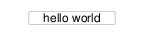In other words, in addition to the convenient high-level interface to the text box, we can now also access a low-level interface to the individual components of the text box.

## The grid.reorder() function

Another new grid function in R 2.16.0 is the grid.reorder() function. This function allows reordering of the children of a gTree. In order to demonstrate the importance of the ordering of grobs, the following code modifies the box component of the current scene so that it is filled grey.
```> grid.edit("box", gp=gpar(fill="grey"))
```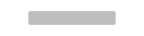The unfortunate result is that the text disappears. This happens because the children of the gTree "tbt" are in the order "text first followed by box", so the box is drawn on top of the text. The grid.reorder() function allows us to fix this sort of thing by changing the order of the children. For example, the following code sends the child "box" to the back.
```> grid.reorder("tbt", "box")
```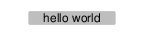A listing of the grobs in the scene shows the new order, with the text now drawn after the box.
```> grid.ls(fullNames=TRUE)
```
```forcedgrob[tbt]
forcedgrob[box]
text[text]
```
The first argument to the grid.reorder() function identifies a gTree in the current scene and the second argument specifies a new ordering for the children of that gTree. The example above demonstrates that it is not necessary to name all children in the reordering; by default, the children named are used first in the new ordering and then all subsequent children are used. For example, the following code would produce the same result.
```> grid.reorder("tbt", c("box", "text"))
```
A third argument, back, can be set to FALSE, in which case the unnamed children are used first, followed by the children named in the second argument, to produce a new ordering.

## The grid.revert() function

One downside of calling grid.force() is that the convenient high-level interface to a custom grob is no longer available. For example, changing the label on the text box no longer has any effect.
```> grid.edit("tbt", label="test")
```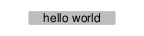The new grid.revert() function is provided to reverse the effect of grid.force() and replace the individual components of the custom grob with the original grob. The following code and shows this function in action. It also demonstrates that the reversion will lose any changes that were made to any of the individual components. We return to the scene that we had before the call to grid.force().
```> grid.revert()
```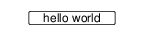In other words, for grobs that generate content at drawing time, we can have either the high-level interface or the low-level interface, but not both at once.

## A reminder

All of the discussion in this article applies to custom grid grobs that need to calculate what to draw at drawing time. If the entire content of a grob or gTree can be generated at creation time, rather than having to wait until drawing time, then things are much easier, and it is possible to have both a high-level interface and low-level access both at the same time. It is only when the content must be generated at drawing time that the design decisions and functions described in this article become necessary.

## A more complex example

The ggplot2 package , via the gtable package , relies on grid grobs that generate their content at drawing time. For example, the following code uses the qplot() function from the ggplot2 package to draw a plot (see Figure
```> library(ggplot2)
```
```> qplot(mpg, wt, data=mtcars, colour=cyl)
```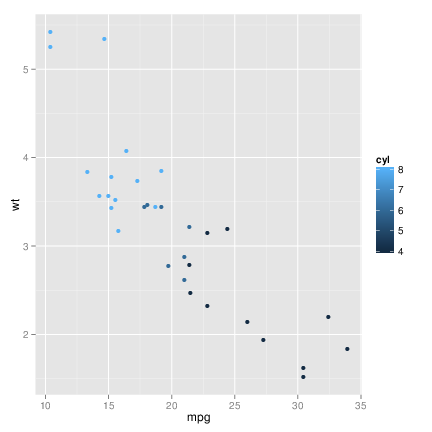A plot produced by the qplot() function from the ggplot2 package.

This entire plot is represented by a single grob, which generates the content to draw the plot at drawing time.
```> grid.ls(fullNames=TRUE)
```
```gtable[layout]
```
It is possible to modify many features of the plot via the ggplot2 theming system, but there is no direct access to the low-level grobs that are generated at drawing time to draw the plot. However, we can gain access to the low-level grobs using grid.force().
```> grid.force()
```
This does not change the appearance of the plot, but the low-level grobs that draw the plot are now visible.
```> grid.ls(fullNames=TRUE)
```
```forcedgrob[layout]
forcedgrob[background.1-6-6-1]
forcedgrob[spacer.4-3-4-3]
forcedgrob[panel.3-4-3-4]
gTree[grill.gTree.147]
rect[panel.background.rect.138]
polyline[panel.grid.minor.y.polyline.140]
polyline[panel.grid.minor.x.polyline.142]
polyline[panel.grid.major.y.polyline.144]
polyline[panel.grid.major.x.polyline.146]
points[geom_point.points.134]
zeroGrob[panel.border.zeroGrob.135]
forcedgrob[axis-l.3-3-3-3]
zeroGrob[axis.line.y.zeroGrob.157]
forcedgrob[axis]
forcedgrob[axis.1-1-1-1]
forcedgrob[axis.1-2-1-2]
forcedgrob[axis-b.4-4-4-4]
zeroGrob[axis.line.x.zeroGrob.151]
forcedgrob[axis]
forcedgrob[axis.1-1-1-1]
forcedgrob[axis.2-1-2-1]
forcedgrob[xlab.5-4-5-4]
forcedgrob[ylab.3-2-3-2]
forcedgrob[guide-box.3-5-3-5]
forcedgrob[guides.2-2-2-2]
forcedgrob[background.1-6-5-1]
forcedgrob[bar.4-2-4-2]
forcedgrob[label.4-4-4-4]
forcedgrob[title.2-5-2-2]
forcedgrob[ticks.4-2-4-2]
forcedgrob[title.2-4-2-4]
```
One interesting thing that we can do now that we have low-level access is to insert a new grob within this hierarchy of existing grobs. The following code does this by adding a rectangle as a child of the "guide-box" gTree (the legend or key to the right of the plot).
```> grid.add("guide-box", grep=TRUE,
+          rectGrob(gp=gpar(col=NA, fill="grey90"),
+                   name="guide-bg"))
```
If we just list the contents of this "guide-box" we can see the rectangle grob that we have added. Because this new child is the last child, it is drawn after the other contents of the legend (i.e., on top of the other contents of the legend; see Figure
```> grid.ls(grid.get("guide-box", grep=TRUE),
+         fullNames=TRUE)
```
```forcedgrob[guide-box.3-5-3-5]
forcedgrob[guides.2-2-2-2]
forcedgrob[background.1-6-5-1]
forcedgrob[bar.4-2-4-2]
forcedgrob[label.4-4-4-4]
forcedgrob[title.2-5-2-2]
forcedgrob[ticks.4-2-4-2]
rect[guide-bg]
```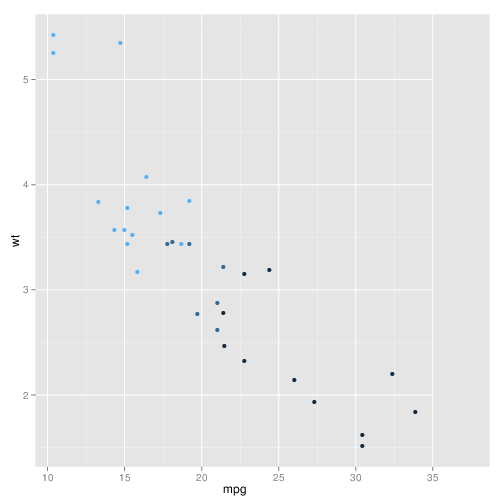The plot from Figure link with a rectangle added to (on top of) the tree of grobs that represent the legend on the right of the plot.

The following code uses grid.reorder() to fix up the order of the grobs in the "guide box" so that the new rectangle is at the back.
```> grid.reorder("guide-box", "guide-bg", grep=TRUE)
```
A listing of the grobs in the "guide box" now shows the added rectangle at the start, which means it will be drawn behind the other content in the legend (see Figure link).
```> grid.ls(grid.get("guide-box", grep=TRUE),
+         fullNames=TRUE)
```
```forcedgrob[guide-box.3-5-3-5]
rect[guide-bg]
forcedgrob[guides.2-2-2-2]
forcedgrob[background.1-6-5-1]
forcedgrob[bar.4-2-4-2]
forcedgrob[label.4-4-4-4]
forcedgrob[title.2-5-2-2]
forcedgrob[ticks.4-2-4-2]
```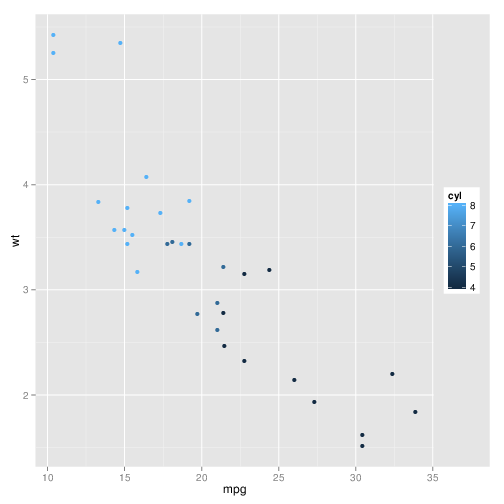The plot from Figure link with the rectangle shifted to the back of the tree of grobs that represent the legend on the right of the plot.

## The new makeContext() hook

Another situation that leads to developing a custom grob occurs when the drawing context for a grob has to be determined at drawing time. In this situation, rather than generating grobs at drawing time, viewports must be generated at drawing time. The old solution for this situation was to write a preDrawDetails() method to generate and push one or more viewports (and a postDrawDetails() method to clean up again after drawing). The new solution is to use the makeContext() function to just generate one or more viewports (and grid automatically takes care of pushing the viewports and cleaning up afterwards). To demonstrate the new approach, we will reimplement the "boxed label" example again. The textbox() function will remain the same as before, just generating a "textboxtree".
```> textbox <- function(label,
+                     name=NULL, gp=NULL, vp=NULL) {
+     grid.draw(gTree(label=label,
+                     name=name, gp=gp, vp=vp,
+                     cl="textboxtree"))
+ }
```
However, we will create a makeContext() method that sets up a viewport to draw the text and box within. This viewport is sized appropriately for the text label and is combined with any existing viewports in the vp slot of the object. It is important that the method returns the modified object.
```> makeContext.textboxtree <- function(x) {
+     tbvp <-
+         viewport(width=1.5*stringWidth(x\$label),
+                  height=1.5*stringHeight(x\$label))
+     if (is.null(x\$vp))
+         x\$vp <- tbvp
+     else
+         x\$vp <- vpStack(x\$vp, tbvp)
+     x
+ }
```
The makeContent() method becomes simpler now because the box just fills the entire viewport that was set up in the makeContext() method.
```> makeContent.textboxtree <- function(x) {
+     t <- textGrob(x\$label, name="text")
+     rr <- roundrectGrob(name="box")
+     setChildren(x, gList(t, rr))
+ }
```
The following code shows that the textbox() function produces exactly the same output as before.
```> grid.newpage()
> textbox("test", name="tbt")
```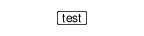Because the drawing context is generated at drawing time, modifying the text label updates the viewport that both text and box are drawn in, so the box expands with the text.
```> grid.edit("tbt", label="hello world")
```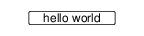## Another reminder

Modifying the drawing context at drawing time is not always necessary. When creating a custom grob, it is often simpler just to set up the drawing context at creation time by creating childrenvp for the children of a gTree. It is only when the generation of drawing context has to be delayed until drawing time that a makeContext() method becomes necessary.

## When to use makeContent() or makeContext()

These functions are only necessary when it is not possible to determine either the drawing context or the drawing content at creation time. These functions may be used in other situations. For example, the main example used in this article does not strictly require using makeContent() because editDetails() could be used instead. So a developer could choose to use makeContent() in some cases because it may be easier than writing a editDetails() method. One example where makeContent() is necessary is the roundrect grob, which must perform conversion of locations to inches to determine what to draw and that calculation needs to be done at drawing time to make sure that it will work in any drawing context.\footnote{This is why the grob listing on page \pageref{page:force} shows a forcedgrob for the box; grid.force() replaced the roundrect with the polygon that the roundrect generated at drawing time in its makeContent() method.} Another example is axis grobs when there is no explicit specification of tick mark locations. In that case, the axis grob must wait until drawing time to know the native scale of the viewport it is being drawn in. Only then can the axis grob determine an appropriate set of tick mark locations. Finally, the gtable example demonstrates that a grob may wish to delay construction of its content until drawing time because the grob is expected to undergo dramatic changes before drawing occurs. In this case, it is inefficient to update the contents of the grob every time it is modified; it is better to wait until drawing time and perform the construction only once. These are only examples of situations that might motivate the use of makeContext() and makeContent(). In some cases, the decision will be forced, but in other cases the choice may be deliberate, so there is no fixed rule for when we might need to use these functions. It is also important to remember that simpler options may exist because grid already delays many calculations until drawing time via the use of gp and vp slots on grobs and the use of units for locations and dimensions. While it would be wrong to characterise these functions as a "last resort", developers of custom grobs should think at least twice before deciding that they are the best solution.

## Summary

The functions makeContext() and makeContent() provide a new approach to developing custom grid grobs (replacing the old approach based on drawDetails() and preDrawDetails()). The advantage of the new approach is that, for grobs that generate content at drawing time, it is possible to access and edit the low-level content that is generated at drawing time by calling the grid.force() function. Another new function, grid.reorder(), allows the modification of the order of the children of a gTree. Together, these new features allow for greater flexibility in the development of custom grid grobs and greater powers to access and modify the low-level details of a grid scene.

## Availability

At the time of writing, the new grid functions makeContext(), makeContent(), grid.force(), grid.revert(), and grid.reorder() are only available in the development version of R. They will be part of the R version 2.16.0 release. The ggplot2 examples depend on a fork of the gtable package with a new implementation based on makeContext() and makeContent(), which is available at github (link). A more technical document decsribing the development and testing of these changes is available from the R developer web site (link).

Paul Murrell
Department of Statistics
The University of Auckland
New Zealand
paul@stat.auckland.ac.nz

## Bibliography

ggplot2 : Elegant graphics for data analysis , 2009,

gtable : Arrange grobs in tables. , 2012, R package version 0.1.1.99